• Hough变换之Hough直线检测
2018-11-03 14:31:28

原创文章，保留所有版权。转载请注明出处：http://www.letao.ai/?p=282

Hough变换的主要思想是，基于已知边缘点的，对所有可能的参数空间中的参数进行投票。在正确的参数取值处，形成峰值，最终得到要求的结果。常用的基于Hough变换检测的有，Hough直线检测、Hough圆检测，和广义Hough变换。

# Hough直线检测

直线如下图所示：Hough直线检测，是将直线方程变换到极坐标下，将求k、b的问题，转换为求取θ、ρ。

具体过程为，离散化θ、ρ，如θ取值为[0:360),  ρ取值为[-200:200]。然后对检测到的每个边缘点，对θ、ρ进行投票。则在有直线的地方，会形成投票峰值。从而得到θ、ρ，进一步得到直线方程。完成直线检测。最后可以通过最小二乘类似的方法，对直线方程进行拟合，提高所求直线的精度。Hough变换
更多相关内容
• 利用matlab检测图像，首先进行滤波，二值化，后采用边缘检测出边缘直线，利用Hough变换检测直线，计算直线之间的像素距离。可以直接在MATLAB中使用；
• Python 基于 hough 直线检测的倾斜图片校正。 基于hough直线检测的倾斜图片校正。 可用Python语言实现基于hough直线检测的倾斜图片校正，内配图片 hough变换
• 利用Hough变换完成直线检测，网上的huogh检测程序总是出错，我的这个程序已经调试通过，放心下载.直线检测的流程是：输入灰度图像，接下来是二值化处理变成黑白图像，然后通过Canny边缘检测算子获取边缘，最后利用...
• import cv2 import numpy as np # 展示图像，封装成函数 def cv_show_image(name, img): cv2.imshow(name, img) ...# 2.canny边缘检测，获取图像边缘信息。减少计算量 # 3.获取霍夫直线信息，可以使用HoughLines ..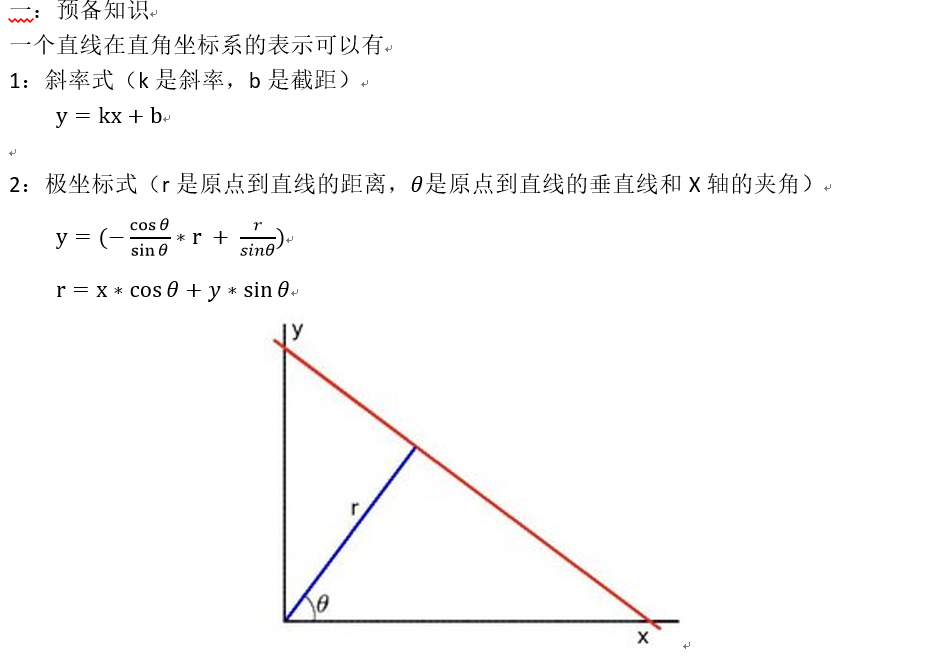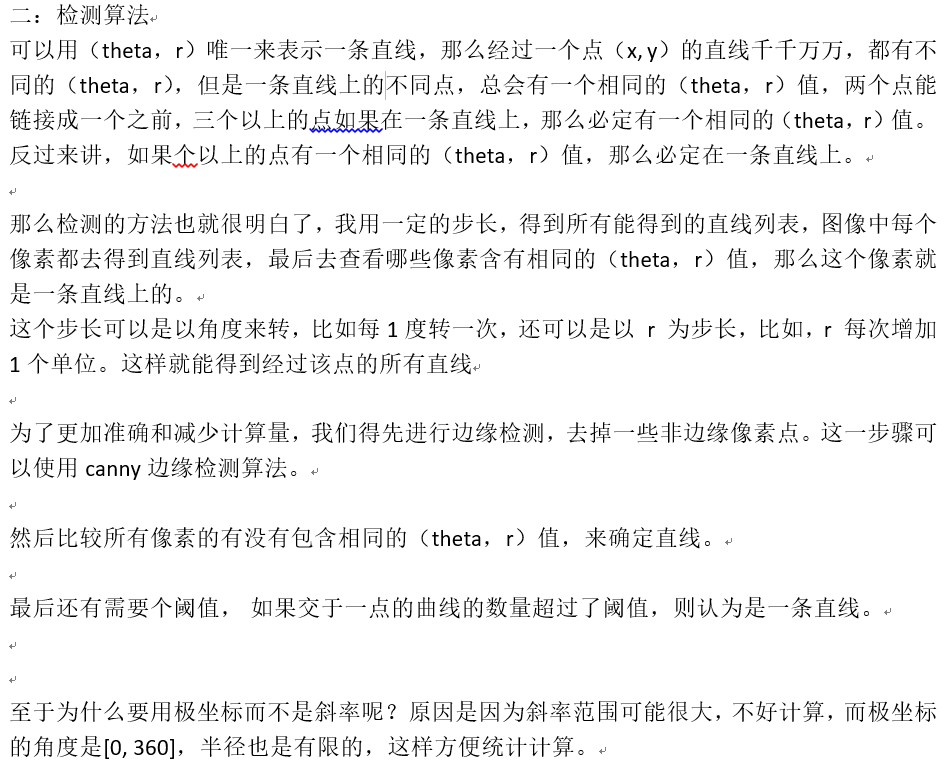import cv2
import numpy as np

# 展示图像，封装成函数
def cv_show_image(name, img):
cv2.imshow(name, img)
cv2.waitKey(0)  # 等待时间，单位是毫秒，0代表任意键终止
cv2.destroyAllWindows()

# 总流程
# 1.获取灰度图像，转成二值图像
# 2.canny边缘检测，获取图像边缘信息。减少计算量
# 3.获取霍夫直线信息，可以使用HoughLines 或者 HoughLinesP函数
# 4.算出直线位置，根据不同的使用函数，获取相应的值，画出每条直线

# 第一步：读取图像
height = img.shape  # 高度
width = img.shape  # 宽度

# 第二步：转成灰度图和二值图像
gray = cv2.cvtColor(img, cv2.COLOR_BGR2GRAY)
ret, gray = cv2.threshold(gray, 127, 255, cv2.THRESH_BINARY)
cv_show_image('gray_img', gray)

# 第三步：使用canny边缘检测，检测出所有的边缘信息
edges = cv2.Canny(gray, threshold1=20, threshold2=100, apertureSize=3)  # apertureSize 是sobel算子的大小
cv_show_image('canny_img', edges)

# 第四步：使用直线检测，并画出直线。
# 第四步第一种方法：使用HoughLines
# dst:    输出图像. 它应该是个灰度图 (但事实上是个二值化图)
# lines:  返回值：储存着检测到的直线的参数对 (x_{start}, y_{start}, x_{end}, y_{end}) 的容器，结构如[[[x1,y1,x2,y2]],[[...]]]
# rho :   参数极半径 r 以像素值为单位的分辨率. 我们使用 1 像素为步长.
# theta:  参数极角 theta 以弧度为单位的分辨率. 我们使用 1度 (即CV_PI/180)为步长.
# threshold:  控制检测出线段的条件。设置阈值： 一条直线所需最少的的曲线在一点相交，超过设定阈值才被检测出线段，值越大，
#           基本上意味着检出的线段越长，检出的线段个数越少。因为阈值越大，超过阈值的点越少，即xy平面的直线越少，但是因为阈值越大，说明检测出在同一条直线上的点越多，即直线越长。这个参数是针对参数空间而言的，在参数空间中一条曲线就代表在xy平面上的一个点
# minLinLength: 能组成一条直线的最少点的数量. 点数量不足的直线将被抛弃，其实这个参数的意义跟上一个很类似，只是这个参数是针对xy平面而言的，即当组成直线的点达到一点数量的时候才被检测出为线段，否则过滤掉
# maxLineGap:   控制检测出直线长短，在同一条直线上的点，能够被连接的最大距离，越大得到的线越长，如下图，点在直线上，若此时距离maxlineGap小于设定的值，则连接，否则就不连接
lines = cv2.HoughLines(image=edges, rho=1, theta=np.pi / 180, threshold=100)  # #函数将通过步长为1的半径和步长为π/180的角来搜索所有可能的直线
print(lines.shape)

result = img.copy()
for line in lines:
# print(type(line))   #多维数组
rho, theta = line  # 获取极值ρ长度和θ角度
a = np.cos(theta)  # 获取角度cos值
b = np.sin(theta)  # 获取角度sin值
x0 = a * rho  # 获取x轴值
y0 = b * rho  # 获取y轴值　　x0和y0是直线的中点
x1 = int(x0 + 1000 * (-b))  # 获取这条直线最大值点x1
y1 = int(y0 + 1000 * (a))  # 获取这条直线最大值点y1
x2 = int(x0 - 1000 * (-b))  # 获取这条直线最小值点x2
y2 = int(y0 - 1000 * (a))  # 获取这条直线最小值点y2　　其中*1000是内部规则
cv2.line(result, (x1, y1), (x2, y2), (0, 0, 255), 2)  # 开始划线
cv_show_image('HoughLines_result_img', result)

# 第四步方法二：使用HoughLinesP
# HoughLinesP概率霍夫变换（是加强版）使用简单，效果更好，检测图像中分段的直线（而不是贯穿整个图像的直线）
minLineLength = 30  # height/32
maxLineGap = 10  # height/40
lines = cv2.HoughLinesP(image=edges, rho=1, theta=np.pi / 180, threshold=80,
minLineLength=minLineLength, maxLineGap=maxLineGap)
print(lines.shape)

result = img.copy()
for line in lines:
# print(type(line))  # 多维数组
x1,y1,x2,y2 = line
cv2.line(result, (x1, y1), (x2, y2), (0, 0, 255), 2)  # 开始划线
cv_show_image('HoughLinesP_result_img', result)



效果展示
二值图像：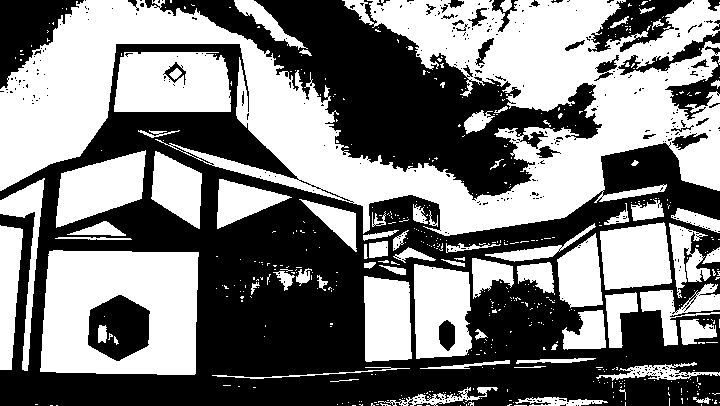canny边缘检测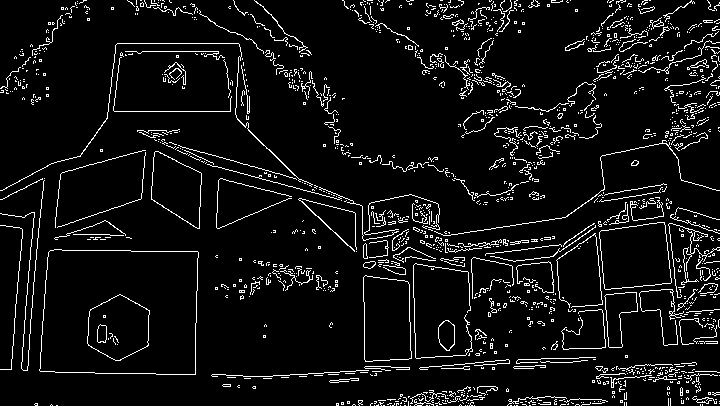使用 HoughLines 函数得到的结果，发现这个直线会穿过整个图像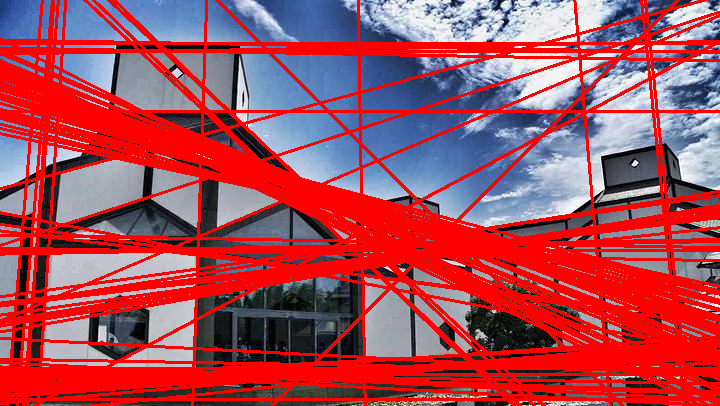使用 HoughLinesP 函数得到的结果，发现这个直线就是图像中的直线长度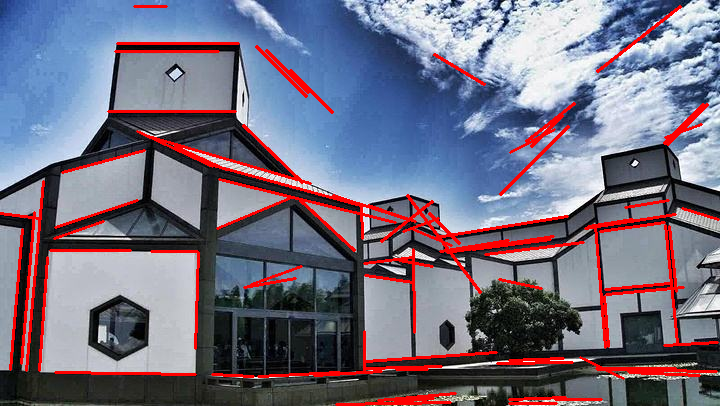最后是参考的资料地址
参考：
霍夫（Hough）变换之直线检测

OpenCV—直线检测

霍夫线变换

展开全文opencv 计算机视觉 学习
• 主要为大家详细介绍了Java+opencv3.2.0之hough直线检测，具有一定的参考价值，感兴趣的小伙伴们可以参考一下
• 简单的讲一下原理，我们知道在两点可以确定一条直线，过一点有无数条的直线，我们在这里给出角度的量化等级为360个等级，那么可以知道的是过一点会有360条直线（重叠的不算同一条）
• 主要介绍了python hough变换检测直线的实现方法，文中通过示例代码介绍的非常详细，对大家的学习或者工作具有一定的参考学习价值，需要的朋友们下面随着小编来一起学习学习吧
• python+opencv–Hough直线检测 通过Canny算子等边缘检测方法获得图像的边缘信息之后， 我们得到仅是多组连续的边缘像素点， 这些像素点包含了极为有用的信息， 但是这些信息我们无法直接使用， 因为图像噪声和图像...

## python+opencv–Hough直线检测

通过Canny算子等边缘检测方法获得图像的边缘信息之后， 我们得到仅是多组连续的边缘像素点， 这些像素点包含了极为有用的信息， 但是这些信息我们无法直接使用， 因为图像噪声和图像像素误差的存在，图像的边缘轮廓并不是规则的几何线条， 不能直接通过函数来表达。比如从下面的建筑物图片和Canny算子提取的边缘图中，可以明显看到边缘图像中包含了大量建筑物的边缘信息。但是，放大建筑物的边缘图像， 可以发现原来我们主观认为很笔直的建筑， 在图像中并不是笔直的线条，而是曲折的像素点， 如下图所示， 因此边缘检测之后还需要对图像边缘进行特征提取， 从离散的像素点中提取有用的特征信息.# Hough变换

在直线检测中， Hough变换采用参数空间变换的方法提取边缘像素带中直线， Hough变换可以降低噪声的影响，对连续直线的检测具有鲁棒性.在直角坐标系中， 直线的方程为: y = k x + b y = kx + b .
直线方程可以唯一的用 ( k , b ) (k,b) 来表示，也可以用极坐标中 ( ρ , θ ) (ρ,\theta) 唯一的表示。其中， ρ \rho 表示直线垂点到原点的距离， θ \theta 表示 ρ \rho x x 轴的夹角。
直线方程是唯一的,所以直线上所有的点在极坐标系中对应 ( ρ , θ ) (\rho,\theta) 也是唯一的。
而直角坐标系中的一个点 ( x , y ) (x,y) ，过该点的直线可能有无数条，这无数条直线与 x x 轴的夹角取值范围是 [ 0 , 2 π ] [0, 2\pi] ，则直角坐标系中的一个点对应于极坐标系中的一条正弦曲线
ρ = x c o s θ + y s i n θ \rho=xcos\theta + ysin\theta
同一条直线的 ( ρ , θ ) (\rho, \theta) 是唯一的，因此同一条直线上的点在极坐标中的正弦曲线必然相交于一点轮廓边缘像素点在直角坐标系中的二维像素坐标，转换到极坐标中，就可以得到对应的正弦曲线，如果直接统计所有的正弦曲线的交点，我们会得到很多误匹配的直线。
Hough变换方法是通过统计量化的局部空间的正弦曲线交点，数量满足阈值条件的点才会被选做直线拟合点，具体方法为：

• ( ρ , θ ) (\rho, \theta) 空间量化成许多小格子
• 将图像平面直角坐标系中的每一个直线点变换到 ( ρ , θ ) (\rho, \theta) 空间
• 统计每个小格子中的共线点，设置累计阈值 T T ，如果小格子中的共线点数量大于 T T ，则格子中的共线点可以用于拟合直线参数，小于 T T 的格子被认为是非共线点，丢弃

# opencv函数

opencv中提供了两个函数用于Hough直线检测

• cv.HoughLines()

C++:
void cv::HoughLines	(	InputArray 	image, 		# 输入单通道8-bit二值化图像
OutputArray 	lines, 	 # 输出的直线向量，每条直线用2或3个元素向量（ρ，θ）表示
double 	rho, 				# 累加器的像素分辨率，一般取1像素
double 	theta,			# 累加器的角度分辨率（弧度），一般取np.pi/180
int 	threshold,				# 累加器阈值

''' srn用于多尺度Hough变换，粗累加器距离分辨率为rho，
精确累加器的分辨率为rho/srn（提高提取精度），
srn和stn取0的话表示采用经典Hough变换，不使用多尺度Hough变换'''
double 	srn = 0,
double 	stn = 0,									# 同上，针对角度分辨率theta
double 	min_theta = 0, 					# 检测直线的最小角度
double 	max_theta = CV_PI 		# 检测直线的最大角度
)
Python:
lines	=	cv.HoughLines(	image, rho, theta, threshold[, lines[, srn[, stn[, min_theta[, max_theta]]]]]	)

• cv.HoughLinesP()
Hough变换的改进版本——概率Hough变换
C++:
void cv::HoughLinesP	(	InputArray 	image,
OutputArray 	lines,
double 	rho,
double 	theta,
int 	threshold,
double 	minLineLength = 0,	# 最小直线长度
double 	maxLineGap = 0 			# 最大线段间隙
)
Python:
lines	=	cv.HoughLinesP(	image, rho, theta, threshold[, lines[, minLineLength[, maxLineGap]]]	)


# 示例代码

import cv2 as cv
import numpy as np
import matplotlib as plt

filename = "/home/lihoon/code/opencvImg/hough/building.jpg"
img_p = img.copy() #用于显示概率Hough变换的检测结果

if img is None:
else:
# cv.imshow("Source", img)

# image space change from BGR to GRAY
gray = cv.cvtColor(img, cv.COLOR_BGR2GRAY)

# threshold
# _, binary = cv.threshold(img, 200, 255, cv.THRESH_BINARY)
_, binary = cv.threshold(gray, 0, 255, cv.THRESH_OTSU)
# cv.imshow("Threshold", binary)

# edges detection with Canny method
edges = cv.Canny(binary, threshold1=50, threshold2=200)
# cv.imshow("Canny", edges)

# HoughLines()函数
lines = cv.HoughLines(edges, rho = 1, theta = 1 * np.pi/180, threshold=120, srn=0, stn = 0, min_theta=1, max_theta=2)

for i in range(0, len(lines)):
rho, theta = lines[i], lines[i]
a = np.cos(theta)
b = np.sin(theta)
x0 = a*rho
y0 = b*rho
x1 = int(x0 + 1000*(-b))
y1 = int(y0 + 1000*(a))
x2 = int(x0 - 1000*(-b))
y2 = int(y0 - 1000*(a))
cv.line(img, (x1, y1), (x2, y2), (0, 0, 255), 2)

cv.imshow("Hough_line", img)

# HoughLinesP()函数
lines_p = cv.HoughLinesP(edges, rho = 1, theta = np.pi/180, threshold = 50, minLineLength= 30, maxLineGap=10)

for i in range(len(lines_p)):
x_1, y_1, x_2, y_2 = lines_p[i]
cv.line(img_p, (x_1, y_1), (x_2, y_2), (0, 255, 0), 2)

print("code successful!")
cv.imshow("Hough_line_p", img_p)

cv.waitKey(0)
cv.destroyAllWindows()


检测结果如下图所示展开全文opencv python
• 经典的Hough变换是一种常用的检测直线的方法，Hough变换的研究及应用动态：Hough变换于1962年由Paul Hough提出，并在美国作为被发表。它所实现的是一种从图像空间到参数空间的映射关系。由于具有一些明显优点和可贵...
• ## 史上最详细的Hough直线检测

万次阅读 多人点赞 2019-01-09 22:32:02
最后可以检测出两条车道线，但是，本课题的目的是通过提供一张图片，经过图像处理操作，经过算法模型得到违章的车辆情况，所以不能有人为的因素。...Hough直线检测 1.直线坐标参数空间 在图像x−y坐标空间...

之前写过检测车道线的文章：https://blog.csdn.net/u010712012/article/details/84780943
最后可以检测出两条车道线，但是，本课题的目的是通过提供一张图片，经过图像处理操作，经过算法模型得到违章的车辆情况，所以不能有人为的因素。

所以这里再次回顾一下检测直线的算法之——Hough变换。

#### Hough直线检测

##### 1.直线坐标参数空间

在图像x−y坐标空间中，经过点( x i x_{i} , y i y_{i} )的直线表示为：
y i = a x i + b − − − − − ( 1 ) y_{i}=ax_{i}+b -----(1)
其中，参数a为斜率，b为截矩。
通过点( x i x_{i} , y i y_{i} )的直线有无数条，且对应于不同的a和b值。

如果将 x i x_{i} y i y_{i} 视为常数，而将原本的参数a和b看作变量，则式子(1)可以表示为：
b = − x i a + y i − − − − − ( 2 ) b=−x_{i}a+y_{i}-----(2)

这样就变换到了参数平面a−b。这个变换就是直角坐标中对于( x i x_{i} , y i y_{i} )点的Hough变换。

该直线是图像坐标空间中的点( x i x_{i} , y i y_{i} )在参数空间的唯一方程。考虑到图像坐标空间中的另一坐标( x j x_{j} , y j y_{j} )，它在参数空间中也有相应的一条直线，表示为：

b = − x j a + y j − − − − − ( 3 ) b=−x_{j}a+y_{j}-----(3)

这条直线与点( x i x_{i} , y i y_{i} )在参数空间的直线相交于一点( a 0 a_{0} , b 0 b_{0} )，如图所示：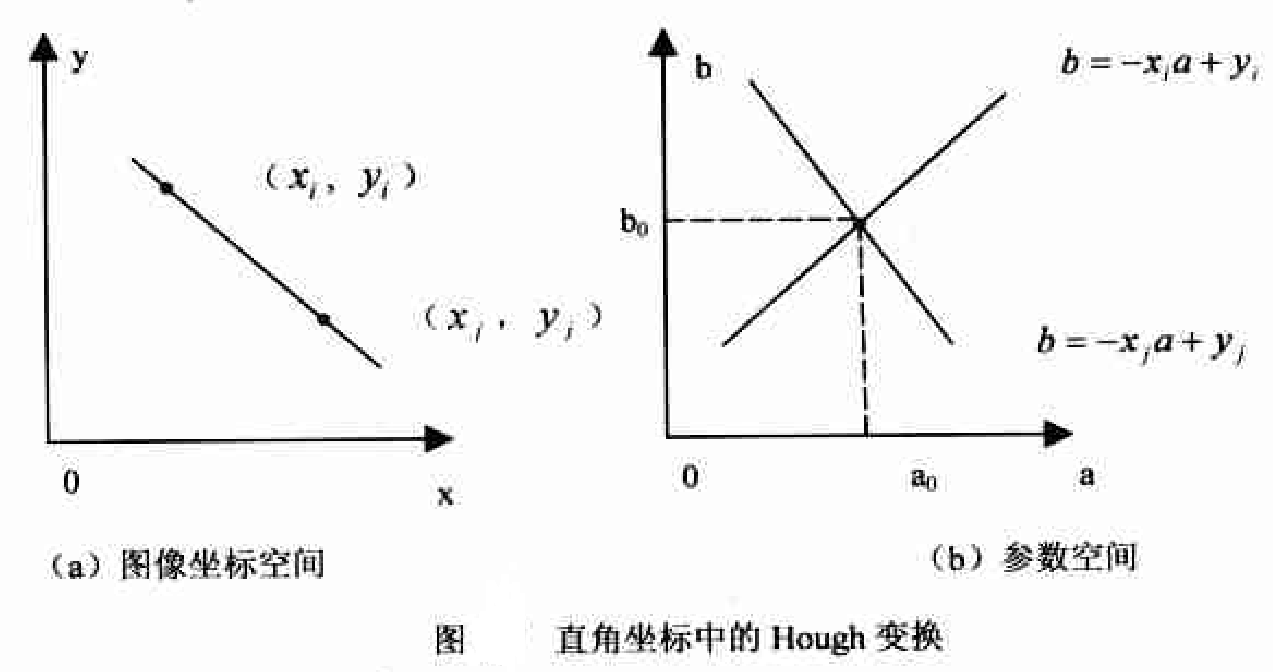图像坐标空间中过点( x i x_{i} , y i y_{i} )和点( x j x_{j} , y j y_{j} )的直线上的每一点在参数空间a−b上各自对应一条直线，这些直线都相交于点 ( a 0 , b 0 ) (a_{0},b_{0}) ,而a0、b0就是图像坐标空间x−y中点( x i x_{i} , y i y_{i} ))和点( x j x_{j} , y j y_{j} )所确定的直线的参数。

反之，在参数空间相交于同一点的所有直线，在图像坐标空间都有共线的点与之对应。根据这个特性，给定图像坐标空间的一些边缘点，就可以通过Hough变换确定连接这些点的直线方程。

具体计算时，可以将参数空间视为离散的。建立一个二维累加数组A(a,b),第一维的范围是图像坐标空间中直线斜率的可能范围，第二维的范围是图像坐标空间中直线截矩的可能范围。开始时A(a,b)初始化为0，然后对图像坐标空间的每一个前景点(xi,yi),将参数空间中每一个a的离散值代入式子(2)中，从而计算出对应的b值。每计算出一对(a,b),都将对应的数组元素A(a,b)加1，即A(a,b)=A(a,b)+1。所有的计算结束之后，在参数计算表决结果中找到A(a,b)的最大峰值，所对应的a0、b0就是原图像中共线点数目最多(共A(a,b)个共线点)的直线方程的参数；接下来可以继续寻找次峰值和第3峰值和第4峰值等等，它们对应于原图中共线点略少一些的直线。

注意：由于原图中的直线往往具有一定的宽度，实际上相当于多条参数极其接近的单像素宽直线，往往对应于参数空间中相邻的多个累加器。因此每找到一个当前最大的峰值点后，需要将该点及其附近点清零，以防算法检测出多条极其邻近的“假”直线。

对于上图的Hough变换空间情况如下图所示。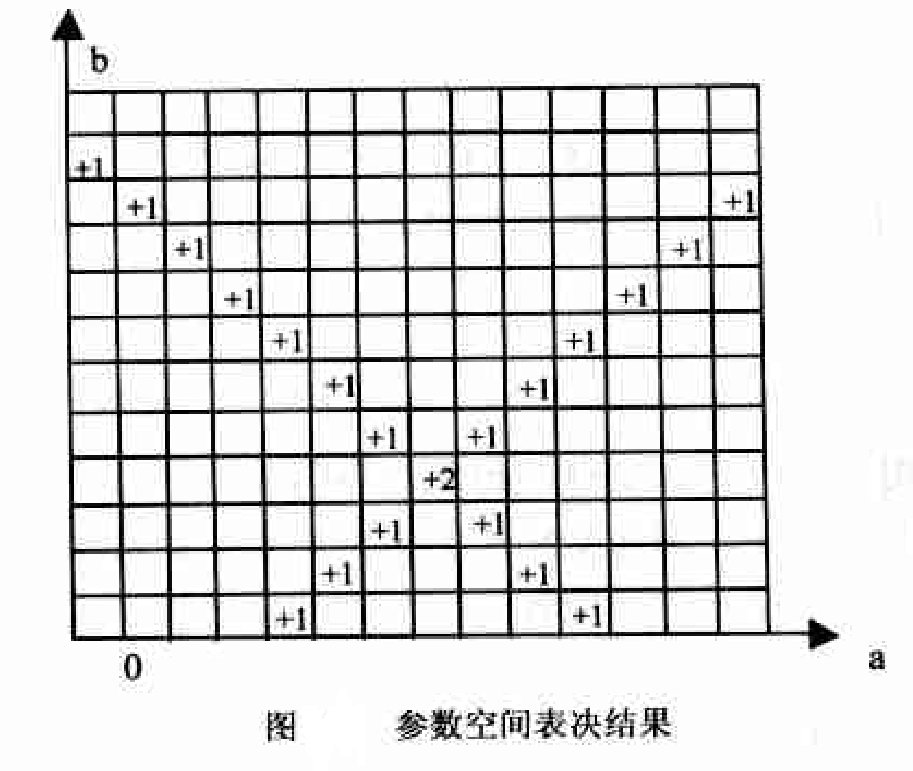这种利用二维累加器的离散方法大大简化了Hough变换的计算，参数空间a−b上的细分程度决定了最终找到直线上点的共线精度。上述的二维累加数组A也被称为Hough矩阵。

##### 2.极坐标参数空间

引入极坐标系的其中一个原因是如果有的直线的斜率不存在，那么直角坐标空间变化就不太合适。

极坐标中用如下参数方程表示一条直线。

ρ = x c o s θ + y s i n θ ( 4 ) ρ=xcosθ+ysinθ(4)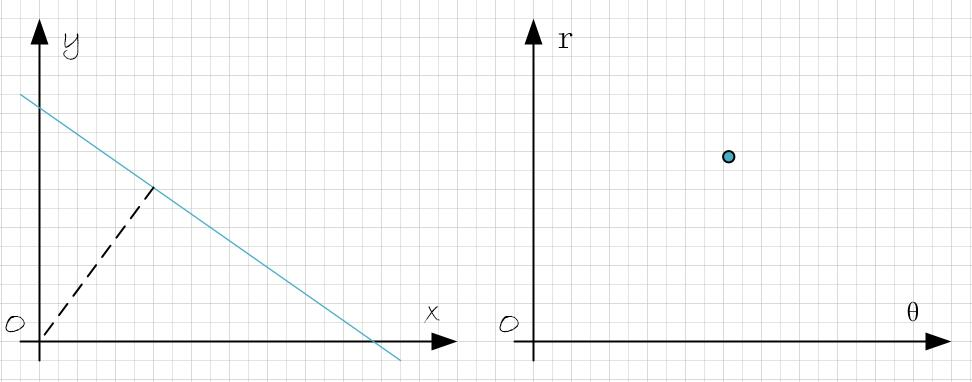其中，ρ代表直线到原点的垂直距离，θ代表x轴到直线垂线的角度，取值范围为±90∘，如图所示。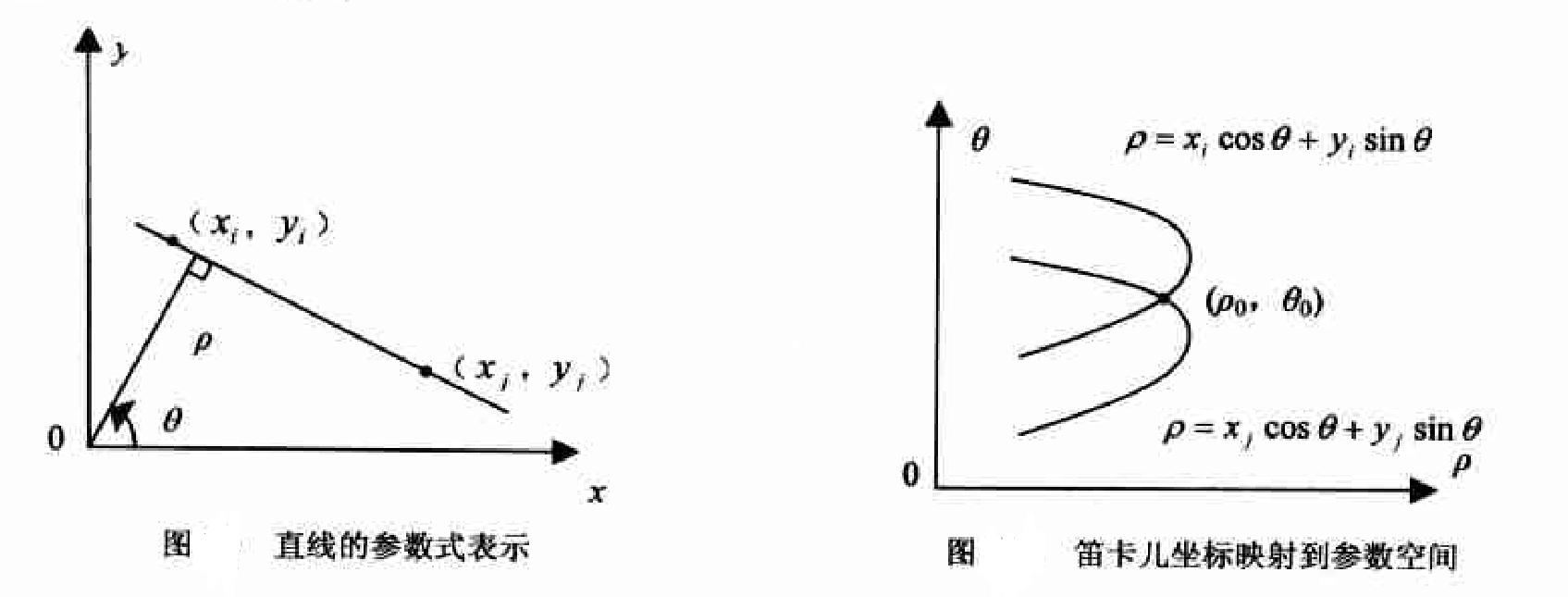与直角坐标类似，极坐标中的Hough变换也将图像坐标空间中的点变换到参数空间中。

更直观的，直角坐标系的三点共线，对应于 ρ − θ \rho-\theta 空间的多线共点。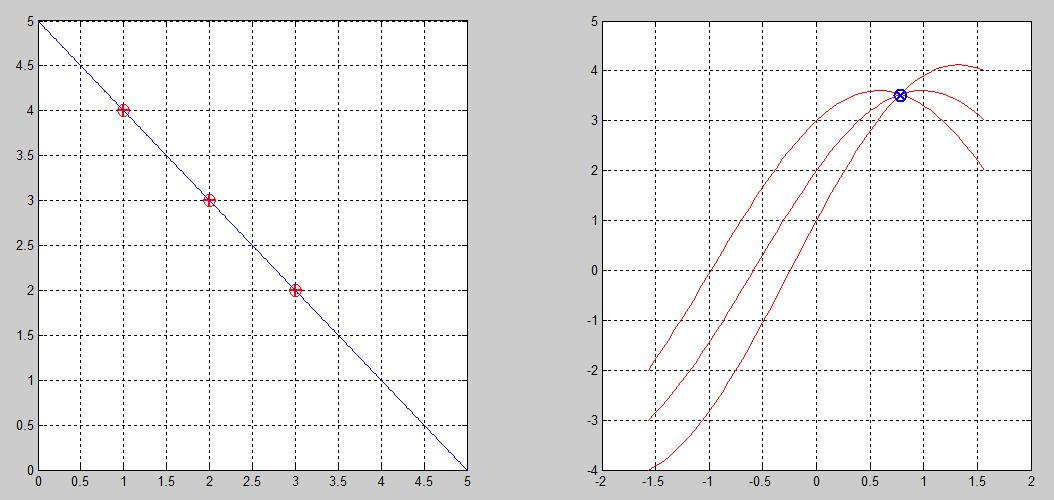上面这个图是如何得到的呢？为什么三点共线在极坐标系是曲线的交点？

我们把直角坐标和极坐标空间融合起来考虑。

在极坐标空间使用hough变换来检测直线的思想就是：为每一个点假设n个方向的直线，通常n=180，此时检测的直线的角度精度为1°，分别计算这n条直线的 ( ρ ， θ (\rho，\theta )坐标，得到n个坐标点。如果要判断的点共有N个，最终得到的 ( ρ ， θ (\rho，\theta )坐标有N * n个。有关这N * n个 ( ρ ， θ (\rho，\theta )坐标，其中theta是离散的角度，共有180个取值。

最重要的地方来了，如果多个点在一条直线上，那么必有这多个点在 θ = \theta= 某个值 θ i \theta_{i} 时，这多个点的 ρ \rho 近似相等于 ρ i \rho_{i} ,也就是说这多个点都在极坐标直线 （ ρ i , θ i ） （\rho_i,\theta_i） 上。

换句话说，在极坐标表示下，原图像坐标空间中共线的点变换到参数空间中后，在参数空间都相交于同一点，此时所得到的ρ、θ即为所求的直线的极坐标参数。与直角坐标不同的是，用极坐标表示时，图像坐标空间的共线的两点(xi,yi)和(xj,yj)映射到参数空间是两条正弦曲线或者参数空间的直线上，相交于点 ( ρ 0 , θ 0 ) (ρ_{0},θ_{0})

下图就更直观了：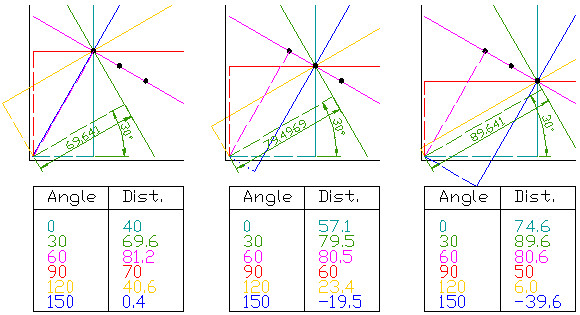这个例子中，对于每个点均求过该点的6条直线的 ( ρ ， θ ) (\rho，\theta) 坐标，共求了3 * 6个 ( ρ ， θ (\rho，\theta )坐标。可以发现在 θ = 60 \theta=60 时，三个点的 ρ \rho 都近似为80.7，由此可判定这三个点都在直线（80.7，60）上。

通过 ρ − θ \rho-\theta 坐标系可以更直观表示这种关系，如下图：图中三个点的（r,theta）曲线汇集在一起，该交点就是同时经过这三个点的直线。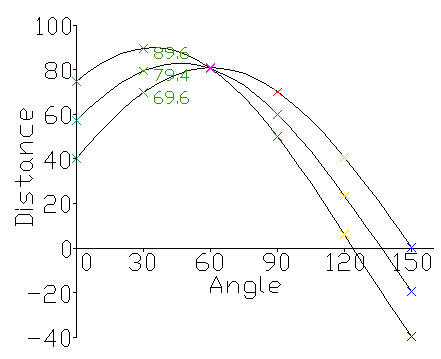在实际的直线检测情况中，如果超过一定数目的点拥有相同的 ( ρ ， θ ) (\rho，\theta) 坐标，那么就可以判定此处有一条直线。在 ρ − θ \rho-\theta 坐标系图中，明显的交汇点就标示一条检测出的直线。

如下图，可以判定出平面上的点共构成了两条直线，即检测出两条直线。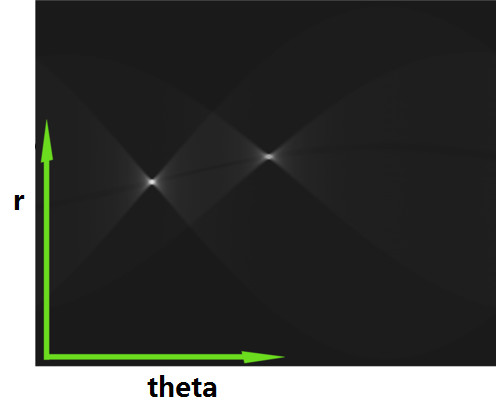因此，我们可以通过检测 ρ − θ \rho-\theta 空间的交集点，来检测原始空间的线段。

接下来，就是要考虑 将 ρ , θ \rho,\theta 离散化，形成离散化的Hough空间，类似于一个矩阵/图像(如下图)，用于统计交集点的个数。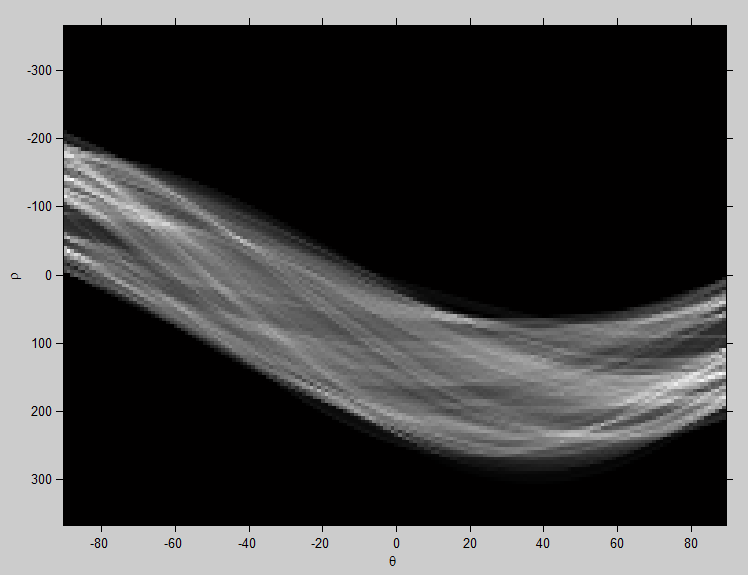具体计算时，与直角坐标类似，也要在参数空间中建立一个二维数组累加器A，只是取值范围不同。对于一副大小为D×D的图像，通常ρ的取值范围为 [ − 2 D / 2 , 2 D / 2 ] , θ [−2\sqrt{D}/2,2\sqrt{D}/2],θ 的取值范围为[−90∘,90∘]。计算方法与直角坐标系中累加器的计算方法相同，最后得到最大的A所对应的(ρ,θ)。

#### 3.Hough变换直线检测的Matlab实现

通过Hough在二值图像中检测直线需要以下3个步骤。
·>(1)利用hough()函数执行霍夫变换，得到霍夫矩阵。
·>(2)利用houghpeaks()函数在霍夫矩阵中寻找峰值点。
·>(3)利用houghlines()函数在之前2步结果的基础上得到原二值图像中的直线信息。

##### 3.1 霍夫变换–Hough

调用形式：
[H,theta,rho]=hough(BW,param1,value1,param2,value2)

参数说明：
·BW是边缘检测后的二值图像；
·可选参数对param1,value1和param2,value2的合法取值如下：

param取值含义
ThetaResolutionHough矩阵中θ轴方向上单位区间的长度(以“度”为单位)，可取(0,90)区间上的实数，默认为1
RhoResolutionHough矩阵中 ρ \rho 轴方向上单位区间的长度，可取(0,norm(size(BW)))区间上的实数，默认为1

返回值：
·H是变换得到的霍夫矩阵
·θ, ρ \rho 分别对应于Hough矩阵每一列和每一行的θ, ρ \rho 值组成的向量。

补充：[m,n] = size(X)

补充：norm的用法，matlab  help norm
NORM   Matrix or vector norm.
For matrices...
对于矩阵...
NORM(X) is the largest singular value of X, max(svd(X)).
NORM(X)是X的最大奇异值
NORM(X,2) is the same as NORM(X).
NORM(X,1) is the 1-norm of X, the largest column sum,
= max(sum(abs(X))).
NORM(X,inf) is the infinity norm of X, the largest row sum,
= max(sum(abs(X'))).
NORM(X,'fro') is the Frobenius norm, sqrt(sum(diag(X'*X))).
NORM(X,P) is available for matrix X only if P is 1, 2, inf or 'fro'.

For vectors...
对于向量...
NORM(V,P) = sum(abs(V).^P)^(1/P).
返回向量A的p范数
NORM(V) = norm(V,2).
返回向量A的2范数，即欧几里德范数。二范数等价于平方和开平方，Sqrt(X1^2+X2^2+...+Xn^2)


##### 3.2 寻找峰值–houghpeaks

调用形式：
peaks=houghpeaks(H,numpeaks,param1,value1,param2,value2)

参数说明：
·H是hough()函数得到的霍夫矩阵
·numpeaks是要寻找的峰值数目，默认为1
·可选参数对param1,value1和param2,value2的合法取值如下：

param取值含义
Threshold峰值的阈值，只有大于该阈值的点才被认为是可能的阈值，取值>0,默认为0.5×max(H())
NHoodSize在每次检测出一个峰值后，NhoodSize指出了在该峰值周围需要清零的领域信息。以[m,n]的形式给出，其中m、n均为正的奇数。默认为大于等于size(H)/50的最小奇数。

返回值：
·peaks是一个Q×2的矩阵，每行的两个元素分别是某一峰值点再hough矩阵中的行、列索引，Q为找到的峰值点的数目。

##### 3.3 提取直线段–houghlines

调用形式：
lines=houghlines(BW,theta,rho,peaks,param1,value1,param2,value2)

参数说明：
·BW是边缘检测后的二值图像
·theta,rho分别对应于Hough矩阵每一列和每一行的θ和ρθ和ρ值组成的向量。有hough()函数返回。
·peaks是一个包含峰值点信息的Q×2Q×2的矩阵，由houghpeaks()函数返回。
·可选参数对param1,value1和param2,value2的合法取值如下：

param取值含义
FillGap线段合并的阈值：如果对应于Hough矩阵某一个单元格(相同的θ和ρ)的两条线段之间的距离小于 FillGap，则合并为一个直线段。默认值为20.
MinLength检测的直线段的最小长度阈值：如果检测出的直线段长度大于MinLength，则保留；丢弃小于MinLength的直线段。默认值为40.

返回值：
·lines是一个结构体数组，数组长度是找到的直线条数，而每一个数组元素(直线段结构体)的内部结构如下：

含义
point1直线段的端点1
point2直线段的端点2
thea对应在霍夫矩阵中的θ
rho对应在霍夫矩阵中的ρ

整体的代码实现

    I  = imread('circuit.tif');
rotI = imrotate(I,33,'crop');
BW = edge(rotI,'canny');
[H,T,R] = hough(BW);
imshow(H,[],'XData',T,'YData',R,'InitialMagnification','fit');
xlabel('\theta'), ylabel('\rho');
axis on, axis normal, hold on;
P  = houghpeaks(H,5,'threshold',ceil(0.3*max(H(:))));
x = T(P(:,2));
y = R(P(:,1));
plot(x,y,'s','color','white');

% Find lines and plot them
lines = houghlines(BW,T,R,P,'FillGap',5,'MinLength',7);
figure, imshow(rotI), hold on
max_len = 0;
for k = 1:length(lines)
xy = [lines(k).point1; lines(k).point2];
plot(xy(:,1),xy(:,2),'LineWidth',2,'Color','green');

% plot beginnings and ends of lines
plot(xy(1,1),xy(1,2),'x','LineWidth',2,'Color','yellow');
plot(xy(2,1),xy(2,2),'x','LineWidth',2,'Color','red');

% determine the endpoints of the longest line segment
len = norm(lines(k).point1 - lines(k).point2);
if ( len > max_len)
max_len = len;
xy_long = xy;
end
end

% highlight the longest line segment
plot(xy_long(:,1),xy_long(:,2),'LineWidth',2,'Color','cyan');


前50个极值点：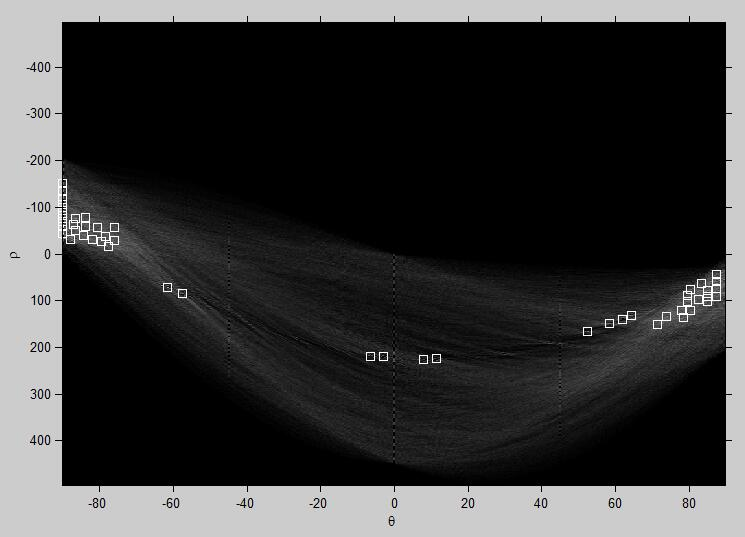这里的Hough空间域就像上面的图，如果随便给我一张图片，我要检测出所有的车道线，那么就是在找Hough参数空间中那个二维累加器中找到峰值，也就是极值，那些极值就是可以被检测原图片的直线，那么将参数空间反映射回图像空间，就能知道哪些地方是直线了，虚线和直线的区别就在于一个阈值，这些参数空间的极值点到底我们选不选，有些极值点是长线段实线，有些极值点可能就是短线段，就是车道虚线，那么我们怎么通过Hough空间的图像判断实线与虚线呢？

展开全文• 基于hough变换的直线检测matlab代码，可直接运行，无需配置
• 利用Opencv中的Houghline方法进行直线检测—python语言 这是给Python部落翻译的文章，请在这里看原文。 在图像处理中，霍夫变换用来检测任意能够用数学公式表达的形状，即使这个形状被破坏或者有点扭曲。 下面我们将...
• ## Hough直线检测的理解

万次阅读 多人点赞 2018-06-02 13:35:51
我们在前面的《图像的颜色选择》、《图像的感兴趣区域》中提到了对车道线的检测。 通过对原始行车图像进行颜色...霍夫变换是1972年提出来的，最开始就是用来在图像中过检测直线，后来扩展能检测圆、曲线等。 我...图像处理
• 1、该代码使用OpenCV进行图片像素的...3、该代码的内容实现是Hough变换的直线检测部分（投票）算法实现 4、该代码使用了Eigen矩阵计算库 5、该代码在Visual Studio2013编译器下编写 6、内含详细代码实现思路及环境搭建
• import cv2 import numpy as np import matplotlib.pyplot as ...img =cv2.imread('E:/python/hough4.jpg') imgray=cv2.cvtColor(img,cv2.COLOR_BGR2GRAY) edge=cv2.Canny(imgray,50,180,apertureSize=3) #Hough直...
• 针对传统的图像配准方法中寻找图像之间点对应关系这一难点问题，提出一种基于Hough直线检测的深度图像配准方法。利用Hough变换检测深度图像上的直线，确定不同视点图像上直线之间的对应关系。根据对应直线三维空间上...
• 可用Python语言实现基于hough直线检测的倾斜图片校正，内配图片
• lines：检测到的直线，一般类型为std::vector<cv::Vec2f> rho: 累加器的距离分辨率（以像素为单位）。 theta：以弧度为单位的累加器的角度分辨率。 threshold：累加器阈值 srn：0 stn：0 min_theta：线条的...opencv
• hough变换检测直线matlab代码直线提取 该算法基于线支持区域概念（Burns'86），该方法首先检测包含具有相似梯度方向的连接像素的区域，然后推断基于线方向和中心位置的检测区域。 主要区别在于我们使用结构张量来...
• 使用MATLAB实现Hough变换，检测车道线的demo程序
•matlab
• import cv2 import numpy as np from matplotlib import pyplot as plt __author__ = ...直线检测 霍夫直线变换 1.霍夫变换(Hough Transform) 霍夫变换是图像处理中从图像中识别几何形状的基本方法之一， ...
• 霍夫线变换是一种在图像中寻找直线的方法。OpenCV中支持三种霍夫线变换，分别是标准霍夫线变换、多尺度霍夫线变换、累计概率霍夫线变换。 在OpenCV中可以调用函数HoughLines来调用标准霍夫线变换和多尺度霍夫线变换...
• matlab实现的hough变换直线检测，单独的.m文件，方便插入调用。matlab
• 网上找到的。想了解Hough霍夫曼直线检测效果的，可以下来学习
• 针对概率Hough变换耗费大量...使用500张图片进行实验验证，算法耗时均低于概率Hough变换耗时的1/3，且对网状聚集边界点的直线错检具有较高抵抗性，检测结果比概率Hough变换直线检测更加准确，内存消耗减少超过90%以上。...